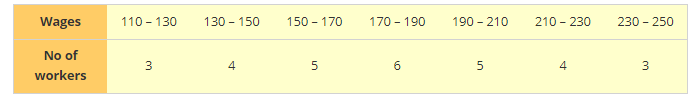# Given below is the frequency distribution of wages (in Rs) of 30 workers in certain factory:

Question:

Given below is the frequency distribution of wages (in Rs) of 30 workers in certain factory:A worker is selected at random. Find the probability that his wages are:

1. Less than Rs.150

2. At least Rs.210

3. More than or equal to 150 but less than 210

Solution:

Total number of workers = 30

1. Probability that the worker wages are less than Rs.150

$=\frac{\text { No of workers having wages below Rs. } 150}{\text { Total no of workers }}$

$=\frac{3+4}{30}=\frac{7}{30}$

2. Probability that the worker wages are at least Rs.210

$=\frac{\text { No of workers having wages below Rs. } 210}{\text { Total no of workers }}$

$=\frac{4+3}{30}=\frac{7}{30}$

3. Probability that the worker wages are more than or equal to 150 but less than 210

$=\frac{\text { No of workers having wages more than Rs. } 150 \text { but less than Rs. } 210}{\text { Total no of workers }}$

$=\frac{5+6+5}{30}=\frac{16}{30}=\frac{8}{15}$